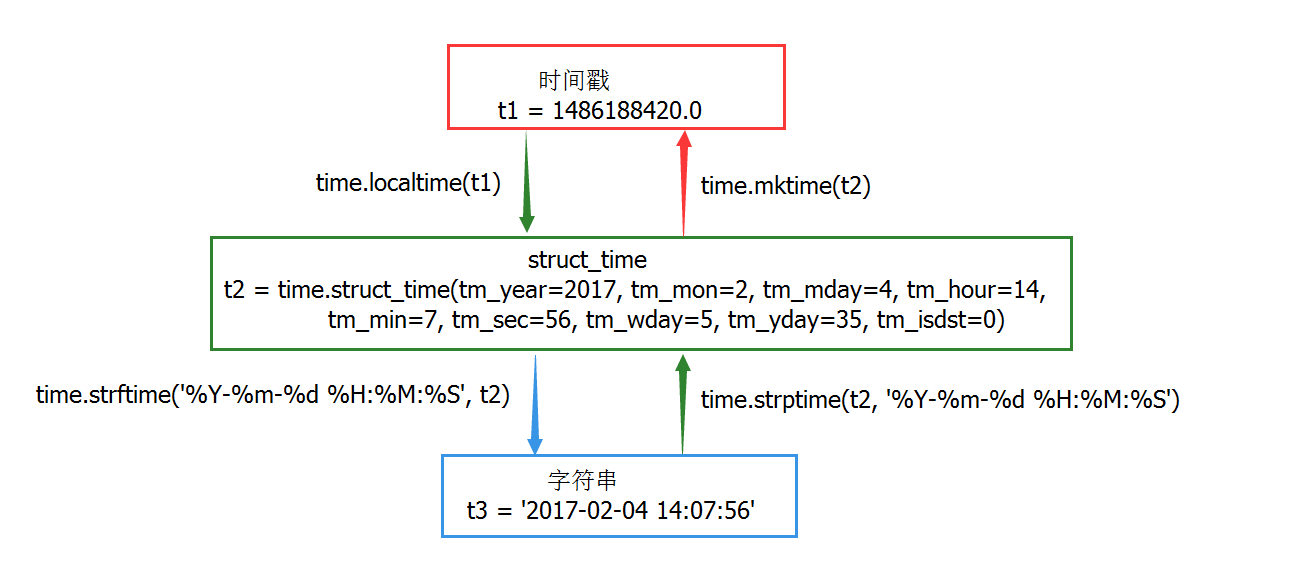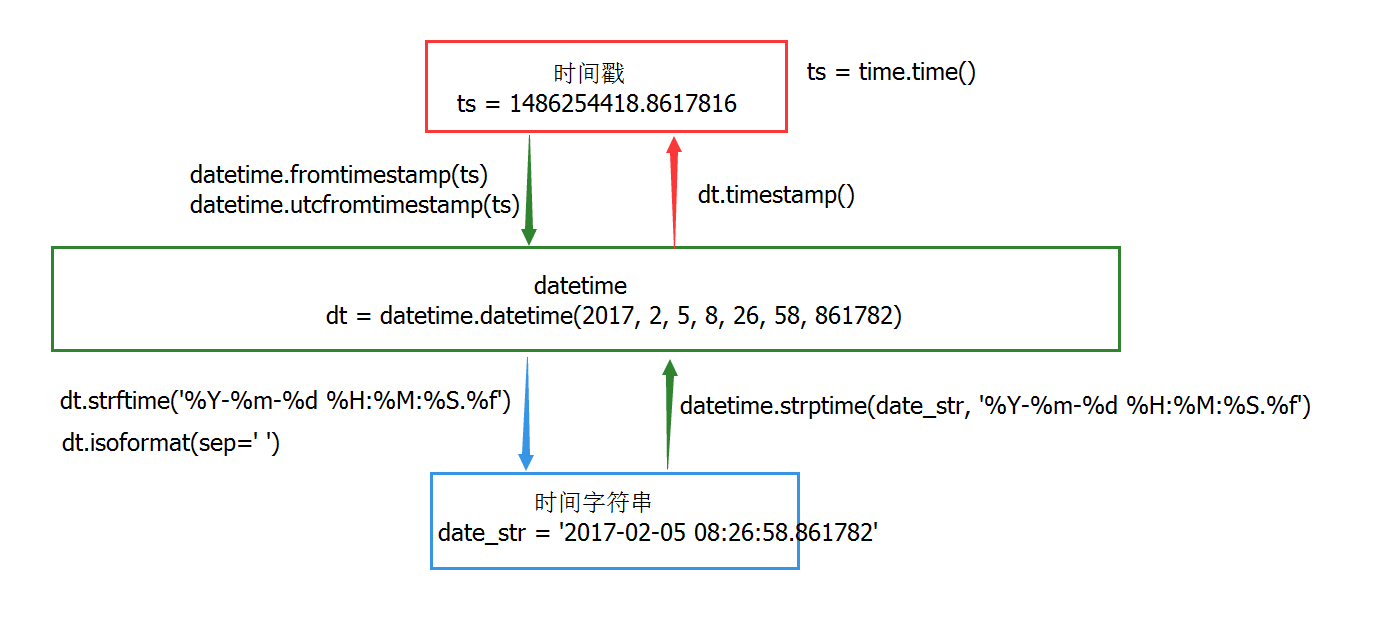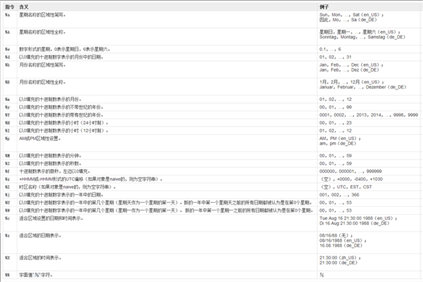# Python之日期与时间处理模块（date和datetime）

Python中提供了多个用于对日期和时间进行操作的内置模块：time模块、datetime模块和calendar模块。其中time模块是通过调用C库实现的，所以有些方法在某些平台上可能无法调用，但是其提供的大部分接口与C标准库time.h基本一致。time模块相比，datetime模块提供的接口更直观、易用，功能也更加强大。

UTC time Coordinated Universal Time，世界协调时，又称 格林尼治天文时间、世界标准时间。与UTC time对应的是各个时区的local time，东N区的时间比UTC时间早N个小时，因此UTC time + N小时 即为东N区的本地时间；而西N区时间比UTC时间晚N个小时，即 UTC time - N小时 即为西N区的本地时间； 中国在东8区，因此比UTC时间早8小时，可以以UTC+8进行表示。 epoch time 表示时间开始的起点；它是一个特定的时间，不同平台上这个时间点的值不太相同，对于Unix而言，epoch time为 1970-01-01 00:00:00 UTC。 timestamp（时间戳） 也称为Unix时间 或 POSIX时间；它是一种时间表示方式，表示从格林尼治时间1970年1月1日0时0分0秒开始到现在所经过的毫秒数，其值为float类型。 但是有些编程语言的相关方法返回的是秒数（Python就是这样），这个需要看方法的文档说明。需要说明的是时间戳是个差值，其值与时区无关。

Python中还有其它的时间表示形式：

time模块的time.struct_time datetime模块的datetime类

time.struct_time包含如下属性：

time模块主要用于时间访问和转换，这个模块提供了各种与时间相关的函数。

1. 函数列表

2. 练习

```>>> time.time()
1486188022.862```

```>>> time.localtime()
time.struct_time(tm_year=2017, tm_mon=2, tm_mday=4, tm_hour=14, tm_min=2, tm_sec=34, tm_wday=5, tm_yday=35, tm_isdst=0)
>>> time.gmtime()
time.struct_time(tm_year=2017, tm_mon=2, tm_mday=4, tm_hour=6, tm_min=2, tm_sec=56, tm_wday=5, tm_yday=35, tm_isdst=0)```

```>>> time.ctime()
'Sat Feb 04 14:06:42 2017'
>>> time.asctime()
'Sat Feb 04 14:06:47 2017'```

```>>> t1 = time.time()
>>> print(t1)
1486188476.9
>>> t2 = time.localtime(t1)
>>> print(t2)
time.struct_time(tm_year=2017, tm_mon=2, tm_mday=4, tm_hour=14, tm_min=7, tm_sec=56, tm_wday=5, tm_yday=35, tm_isdst=0)
>>> t3 = time.gmtime(t1)
>>> print(t3)
time.struct_time(tm_year=2017, tm_mon=2, tm_mday=4, tm_hour=6, tm_min=7, tm_sec=56, tm_wday=5, tm_yday=35, tm_isdst=0)
>>>```

```>>> time.strptime('Sat Feb 04 14:06:42 2017')
time.struct_time(tm_year=2017, tm_mon=2, tm_mday=4, tm_hour=14, tm_min=6, tm_sec=42, tm_wday=5, tm_yday=35, tm_isdst=-1)
>>> time.strptime('Sat Feb 04 14:06:42 2017', '%a %b %d %H:%M:%S %Y')
time.struct_time(tm_year=2017, tm_mon=2, tm_mday=4, tm_hour=14, tm_min=6, tm_sec=42, tm_wday=5, tm_yday=35, tm_isdst=-1)
>>> time.strptime('2017-02-04 14:12', '%Y-%m-%d %H:%M')
time.struct_time(tm_year=2017, tm_mon=2, tm_mday=4, tm_hour=14, tm_min=12, tm_sec=0, tm_wday=5, tm_yday=35, tm_isdst=-1)
>>> time.strptime('2017/02/04 14:12', '%Y/%m/%d %H:%M')
time.struct_time(tm_year=2017, tm_mon=2, tm_mday=4, tm_hour=14, tm_min=12, tm_sec=0, tm_wday=5, tm_yday=35, tm_isdst=-1)
>>> time.strptime('201702041412', '%Y%m%d%H%M')
time.struct_time(tm_year=2017, tm_mon=2, tm_mday=4, tm_hour=14, tm_min=12, tm_sec=0, tm_wday=5, tm_yday=35, tm_isdst=-1)```

struct_time格式转字符串格式时间

```>>> time.strftime('%Y-%m-%d %H:%M', time.localtime())
'2017-02-04 14:19'

struct_time格式转时间戳格式时间
>>> time.mktime(time.localtime())
1486189282.0
```

3. 时间格式转换datetime模块提供了处理日期和时间的类，既有简单的方式，又有复杂的方式。它虽然支持日期和时间算法，但其实现的重点是为输出格式化和操作提供高效的属性提取功能。

1. datetime模块中定义的类

datetime模块定义了以下几个类：

```object
date
datetime
time
timedelta
tzinfo
timezone```

2. datetime模块中定义的常量

3. datetime.date类

datetime.date类的定义

`class datetime.date(year, month, day)`

year, month 和 day都是是必须参数，各参数的取值范围为：

```>>> import time
>>> from datetime import date
>>>
>>> date.max
datetime.date(9999, 12, 31)
>>> date.min
datetime.date(1, 1, 1)
>>> date.resolution
datetime.timedelta(1)
>>> date.today()
datetime.date(2017, 2, 4)
>>> date.fromtimestamp(time.time())
datetime.date(2017, 2, 4)
>>>
>>> d = date.today()
>>> d.year
2017
>>> d.month
2
>>> d.day
4
>>> d.replace(2016)
datetime.date(2016, 2, 4)
>>> d.replace(2016, 3)
>>> d.replace(2016, 3, 2)
datetime.date(2016, 3, 2)
>>> d.timetuple()
time.struct_time(tm_year=2017, tm_mon=2, tm_mday=4, tm_hour=0, tm_min=0, tm_sec=0, tm_wday=5, tm_yday=35, tm_isdst=-1)
>>> d.toordinal()
736364
>>> d.weekday()
5
>>> d.isoweekday()
6
>>> d.isocalendar()
(2017, 5, 6)
>>> d.isoformat()
'2017-02-04'
>>> d.ctime()
'Sat Feb 4 00:00:00 2017'
>>> d.strftime('%Y/%m/%d')
'2017/02/04'
```

4. datetime.time类

time类的定义

`class datetime.time(hour, [minute[, second, [microsecond[, tzinfo]]]])`

hour为必须参数，其他为可选参数。各参数的取值范围为：

```>>> from datetime import time
>>>
>>> time.max
datetime.time(23, 59, 59, 999999)
>>> time.min
datetime.time(0, 0)
>>> time.resolution
datetime.timedelta(0, 0, 1)
>>>
>>> t = time(20, 5, 40, 8888)
>>> t.hour
20
>>> t.minute
5
>>> t.second
40
>>> t.microsecond
8888
>>> t.tzinfo
>>>
>>> t.replace(21)
datetime.time(21, 5, 40, 8888)
>>> t.isoformat()
'20:05:40.008888'
>>> t.strftime('%H%M%S')
'200540'
>>> t.strftime('%H%M%S.%f')
'200540.008888'
```

5. datetime.datetime类

datetime类的定义

`class datetime.datetime(year, month, day, hour=0, minute=0, second=0, microsecond=0, tzinfo=None)`

year, month 和 day是必须要传递的参数， tzinfo可以是None或tzinfo子类的实例。

```>>> from datetime import datetime, timezone
>>>
>>> datetime.today()
datetime.datetime(2017, 2, 4, 20, 44, 40, 556318)
>>> datetime.now()
datetime.datetime(2017, 2, 4, 20, 44, 56, 572615)
>>> datetime.now(timezone.utc)
datetime.datetime(2017, 2, 4, 12, 45, 22, 881694, tzinfo=datetime.timezone.utc)
>>> datetime.utcnow()
datetime.datetime(2017, 2, 4, 12, 45, 52, 812508)
>>> import time
>>> datetime.fromtimestamp(time.time())
datetime.datetime(2017, 2, 4, 20, 46, 41, 97578)
>>> datetime.utcfromtimestamp(time.time())
datetime.datetime(2017, 2, 4, 12, 46, 56, 989413)
>>> datetime.combine(date(2017, 2, 4), t)
datetime.datetime(2017, 2, 4, 20, 5, 40, 8888)
>>> datetime.strptime('2017/02/04 20:49', '%Y/%m/%d %H:%M')
datetime.datetime(2017, 2, 4, 20, 49)
>>> dt = datetime.now()
>>> dt
datetime.datetime(2017, 2, 4, 20, 57, 0, 621378)
>>> dt.year
2017
>>> dt.month
2
>>> dt.day
4
>>> dt.hour
20
>>> dt.minute
57
>>> dt.second
0
>>> dt.microsecond
621378
>>> dt.tzinfo
>>> dt.timestamp()
1486213020.621378
>>> dt.date()
datetime.date(2017, 2, 4)
>>> dt.time()
datetime.time(20, 57, 0, 621378)
>>> dt.timetz()
datetime.time(20, 57, 0, 621378)
>>> dt.replace()
datetime.datetime(2017, 2, 4, 20, 57, 0, 621378)
>>> dt.replace(2016)
datetime.datetime(2016, 2, 4, 20, 57, 0, 621378)
>>> dt.timetuple()
time.struct_time(tm_year=2017, tm_mon=2, tm_mday=4, tm_hour=20, tm_min=57, tm_sec=0, tm_wday=5, tm_yday=35, tm_isdst=-1)
>>> dt.utctimetuple()
time.struct_time(tm_year=2017, tm_mon=2, tm_mday=4, tm_hour=20, tm_min=57, tm_sec=0, tm_wday=5, tm_yday=35, tm_isdst=0)
>>> dt.toordinal()
736364
>>> dt.weekday()
5
>>> dt.isocalendar()
(2017, 5, 6)
>>> dt.isoformat()
'2017-02-04T20:57:00.621378'
>>> dt.isoformat(sep='/')
'2017-02-04/20:57:00.621378'
>>> dt.isoformat(sep=' ')
'2017-02-04 20:57:00.621378'
>>> dt.ctime()
'Sat Feb 4 20:57:00 2017'
>>> dt.strftime('%Y%m%d %H:%M:%S.%f')
'20170204 20:57:00.621378'
```

6. 使用datetime.datetime类对时间戳与时间字符串进行转换7. datetime.timedelta类

timedelta对象表示连个不同时间之间的差值。如果使用time模块对时间进行算术运行，只能将字符串格式的时间 和 struct_time格式的时间对象 先转换为时间戳格式，然后对该时间戳加上或减去n秒，最后再转换回struct_time格式或字符串格式，这显然很不方便。而datetime模块提供的timedelta类可以让我们很方面的对datetime.date, datetime.time和datetime.datetime对象做算术运算，且两个时间之间的差值单位也更加容易控制。

datetime.timedelta类的定义

`class datetime.timedelta(days=0, seconds=0, microseconds=0, milliseconds=0, hours=0, weeks=0)`

1毫秒转换为1000微秒 1分钟转换为60秒 1小时转换为3600秒 1周转换为7天

microseconds : [0, 999999] seconds : [0, 86399] days : [-999999999, 999999999]

```>>> import datetime
>>>
>>> datetime.timedelta(365).total_seconds() # 一年包含的总秒数
31536000.0
>>> dt = datetime.datetime.now()
>>> dt + datetime.timedelta(3) # 3天后
datetime.datetime(2017, 2, 8, 9, 39, 40, 102821)
>>> dt + datetime.timedelta(-3) # 3天前
datetime.datetime(2017, 2, 2, 9, 39, 40, 102821)
>>> dt + datetime.timedelta(hours=3) # 3小时后
datetime.datetime(2017, 2, 5, 12, 39, 40, 102821)
>>> dt + datetime.timedelta(hours=-3) # 3小时前
datetime.datetime(2017, 2, 5, 6, 39, 40, 102821)
>>> dt + datetime.timedelta(hours=3, seconds=30) # 3小时30秒后
datetime.datetime(2017, 2, 5, 12, 40, 10, 102821)
```

time模块的struct_time以及datetime模块的datetime、date、time类都提供了strftime()方法，该方法可 以输出一个指定格式的时间字符串。具体格式由一系列的格式码（格式字符）组成，Python最终调用的是各个平台C库的strftme()函数，因此各平台对全套格式码的支持会有所不同，具体情况需要参考该平台上的strftime(3)文档。下面列出了C标准（1989版）要求的所有格式码，它们在所有标准C实现的平台上都可以工作：Python 遍历列表里面序号和值的方法（三种）

Python爬虫：通过关键字爬取百度图片

Copyright © 2010 Gimoo.Net. All Rights Rreserved  京ICP备05050695号